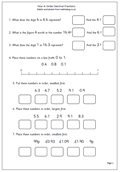# Year 4 maths worksheet on ordering decimal fractionsOne of the most important concepts in maths is to understand that the value of a digit depends on its place in the number – otherwise we could only possibly have 9 numbers and zero! So the digit 2 in 120 has a different value than the 2 in 210. The 2 in 120 has a value of 20, whilst the 2 in 210 has a value of 200.

By Year 4 children should be applying this idea to decimals. The first column to the right of the decimal point is tenths so the 1 in 2.1 has a value of one tenth and the 1 in 2.01 has a value of one hundredth. This is best shown with money: one penny is £0.01, or one hundredth, whilst 10p is £0.10 or one tenth of a pound. (It is also important that we always put two digits after the decimal when using money, but not necessarilyat other times.)

This maths worksheets looks at both place value and ordering simple decimal fractions, using the concepts above.

year 4 worksheet: Order decimal fractions